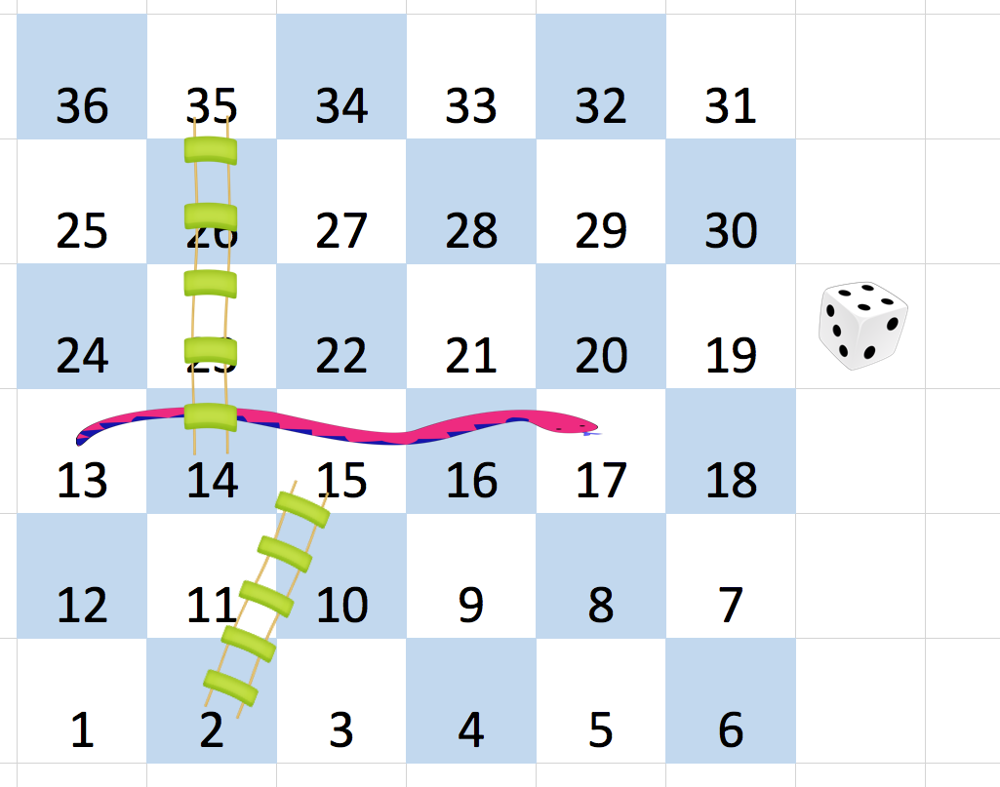## Algorithm

Problem Name: 909. Snakes and Ladders

You are given an `n x n` integer matrix `board` where the cells are labeled from `1` to `n2` in a Boustrophedon style starting from the bottom left of the board (i.e. `board[n - 1]`) and alternating direction each row.

You start on square `1` of the board. In each move, starting from square `curr`, do the following:

• Choose a destination square `next` with a label in the range `[curr + 1, min(curr + 6, n2)]`.
• This choice simulates the result of a standard 6-sided die roll: i.e., there are always at most 6 destinations, regardless of the size of the board.
• If `next` has a snake or ladder, you must move to the destination of that snake or ladder. Otherwise, you move to `next`.
• The game ends when you reach the square `n2`.

A board square on row `r` and column `c` has a snake or ladder if `board[r][c] != -1`. The destination of that snake or ladder is `board[r][c]`. Squares `1` and `n2` do not have a snake or ladder.

Note that you only take a snake or ladder at most once per move. If the destination to a snake or ladder is the start of another snake or ladder, you do not follow the subsequent snake or ladder.

• For example, suppose the board is `[[-1,4],[-1,3]]`, and on the first move, your destination square is `2`. You follow the ladder to square `3`, but do not follow the subsequent ladder to `4`.

Return the least number of moves required to reach the square `n2`. If it is not possible to reach the square, return `-1`.

Example 1:```Input: board = [[-1,-1,-1,-1,-1,-1],[-1,-1,-1,-1,-1,-1],[-1,-1,-1,-1,-1,-1],[-1,35,-1,-1,13,-1],[-1,-1,-1,-1,-1,-1],[-1,15,-1,-1,-1,-1]]
Output: 4
Explanation:
In the beginning, you start at square 1 (at row 5, column 0).
You decide to move to square 2 and must take the ladder to square 15.
You then decide to move to square 17 and must take the snake to square 13.
You then decide to move to square 14 and must take the ladder to square 35.
You then decide to move to square 36, ending the game.
This is the lowest possible number of moves to reach the last square, so return 4.
```

Example 2:

```Input: board = [[-1,-1],[-1,3]]
Output: 1
```

Constraints:

• `n == board.length == board[i].length`
• `2 <= n <= 20`
• `board[i][j]` is either `-1` or in the range `[1, n2]`.
• The squares labeled `1` and `n2` do not have any ladders or snakes.

## Code Examples

### #1 Code Example with Java Programming

```Code - Java Programming```

``````
class Solution {
int n = board.length;
Map map = new HashMap<>();
map.put(1, 0);
while (!queue.isEmpty()) {
int s = queue.remove();
if (s == n * n) {
return map.get(s);
}
for (int i = s + 1; i <= Math.min(s + 6, n * n); i++) {
int rc = helper(i, n);
int r = rc / n;
int c = rc % n;
int point = board[r][c] == -1 ? i : board[r][c];
if (!map.containsKey(point)) {
map.put(point, map.get(s) + 1);
}
}
}
return -1;
}

public int helper(int s, int N) {
int quot = (s - 1) / N;
int rem = (s - 1) % N;
int row = N - 1 - quot;
int col = row % 2 != N % 2 ? rem : N - 1 - rem;
return row * N + col;
}
}
``````
Copy The Code &

Input

cmd
board = [[-1,-1,-1,-1,-1,-1],[-1,-1,-1,-1,-1,-1],[-1,-1,-1,-1,-1,-1],[-1,35,-1,-1,13,-1],[-1,-1,-1,-1,-1,-1],[-1,15,-1,-1,-1,-1]]

Output

cmd
4

### #2 Code Example with Python Programming

```Code - Python Programming```

``````
class Solution:
arr, nn, q, seen, moves = , len(board) ** 2, , set(), 0
for i, row in enumerate(board[::-1]): arr += row[::-1] if i % 2 else row
while q:
new = []
for sq in q:
if sq == nn: return moves
for i in range(1, 7):
if sq + i <= nn and sq + i not in seen:
new.append(sq + i if arr[sq + i] == -1 else arr[sq + i])
q, moves = new, moves + 1
return -1
``````
Copy The Code &

Input

cmd
board = [[-1,-1,-1,-1,-1,-1],[-1,-1,-1,-1,-1,-1],[-1,-1,-1,-1,-1,-1],[-1,35,-1,-1,13,-1],[-1,-1,-1,-1,-1,-1],[-1,15,-1,-1,-1,-1]]

Output

cmd
4